2018-03-26 17:06:07 Julialove102123 阅读数 5325
• ###### OpenCV3.2 Java图像处理视频学习教程

OpenCV3.2 Java图像处理视频培训课程：基于OpenCV新版本3.2.0详细讲述Java OpenCV图像处理部分内容，包括Mat对象使用、图像读写、 基于常用核心API讲述基本原理、使用方法、参数、代码演示、图像处理思路与流程讲授。主要内容包括opencv像素操作、滤波、边缘提取、直线与圆检测、形态学操作与分水岭、图像金子塔融合重建、多尺度模板匹配、opencv人脸检测、OpenCV跟Tomcat使用实现服务器端图像处理服务。

4257 人正在学习 去看看 贾志刚## 中值滤波## 最大最小值滤波## 双边滤波## 引导滤波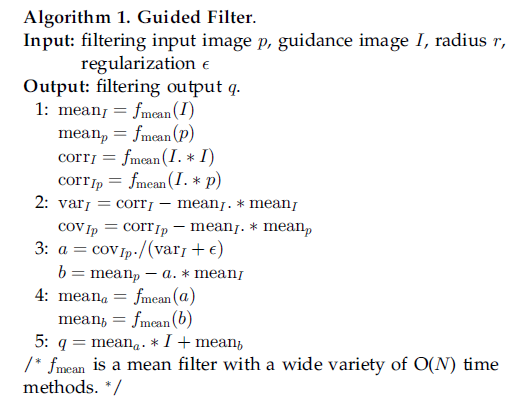def guidedfilter(I,p,r,eps):
'''I:引导图图；
p:输入图（p=I）;
r :半径:
eps:regulation
f:为窗口半径为r的均值滤波器；
corr：相关；
var：方差；
cov：协方差
'''
height,width = I.reshape()
m_I = cv2.boxFilter(I,-1,(r,r))  #f_mean(I) 均值滤波blur和盒式滤波一样
m_p = cv2.boxFilter(p,-1,(r,r))  #f_mean(p)

m_II = cv2.boxFilter(I*I,-1,(r,r)) #f_mean(I.*I)
m_Ip = cv2.boxFilter(I * p, -1, (r, r))  #f_mean(I.*p)

var_I = m_II-m_I*m_I   #求方差：corr_I -mean_I.*mean_I
cov_Ip = m_Ip - m_I * m_p  #协方差： #cov_Ip-mean_I.*mean_p

a = cov_Ip/(var_I+eps)  #cov_Ip./(var_I+eps)
b = m_p-a*m_I   #mean_p -a.*mean_I
m_a = cv2.boxFilter(a,-1,(r,r))  #mean_a
m_b = cv2.boxFilter(b,-1,(r,r))  #mean_b
return m_a*I+m_b

2019-09-08 22:17:24 webzhuce 阅读数 742
• ###### OpenCV3.2 Java图像处理视频学习教程

OpenCV3.2 Java图像处理视频培训课程：基于OpenCV新版本3.2.0详细讲述Java OpenCV图像处理部分内容，包括Mat对象使用、图像读写、 基于常用核心API讲述基本原理、使用方法、参数、代码演示、图像处理思路与流程讲授。主要内容包括opencv像素操作、滤波、边缘提取、直线与圆检测、形态学操作与分水岭、图像金子塔融合重建、多尺度模板匹配、opencv人脸检测、OpenCV跟Tomcat使用实现服务器端图像处理服务。

4257 人正在学习 去看看 贾志刚

## 基本概念

在图像处理中，高斯滤波一般用高斯模板。除此之外，还有递归高斯滤波、FFT法、重复卷积法等。

## 1、直接卷积法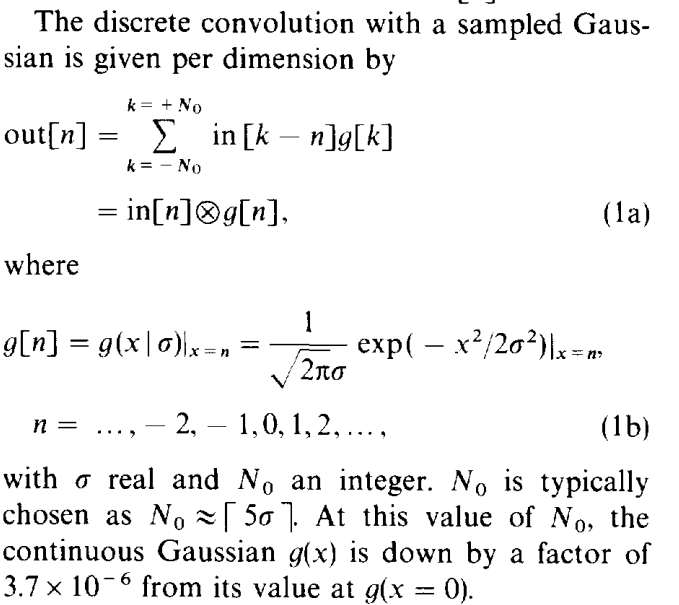## 2、重复卷积法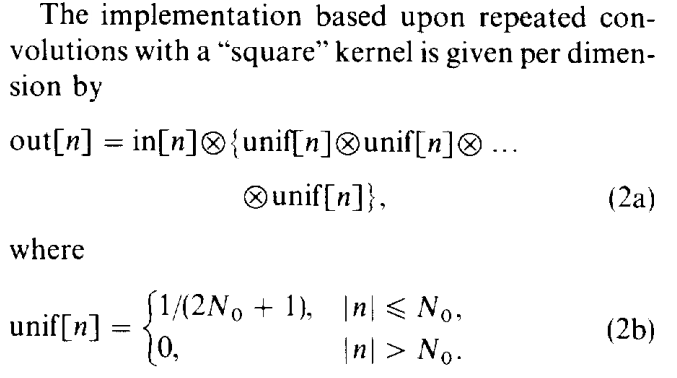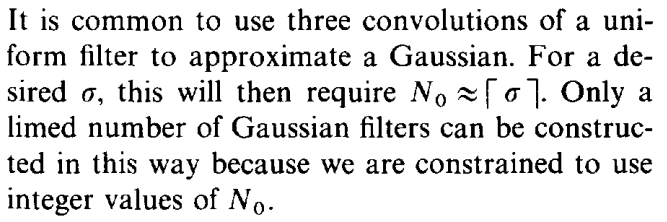## 3、FFT实现法

原理很简单out = IFFT2（FFT（in）*FFT（h）），其中in是输入图像，h为卷积核，out是平滑后图像。

## 4、递归实现法

文献《Recursive implementation of the Gaussian filter》提出该方法，其产生一个IIR滤波器，比上面三种方法都快，其运行时间与σ无关。推导过程大致是，将高斯公式g(t)的傅里叶变换G(w)进行泰勒公式展开，阶数到6取近似,将其拉普拉斯变换G(s),在变换到z域时，用前后差分技术代替双线性法。最终结论如下。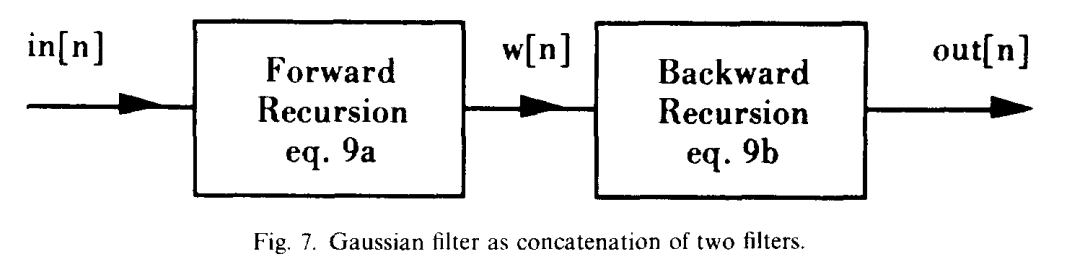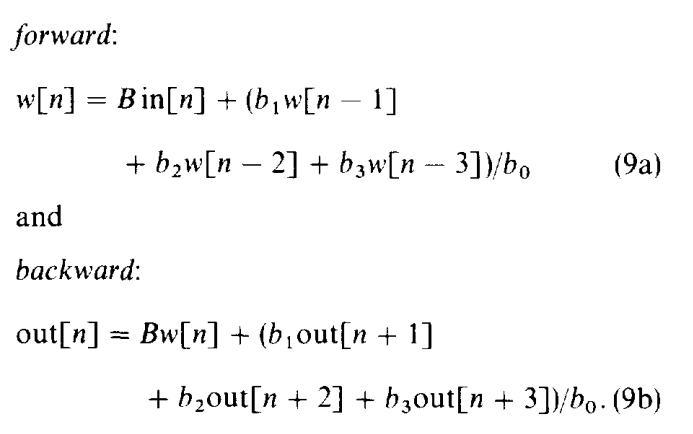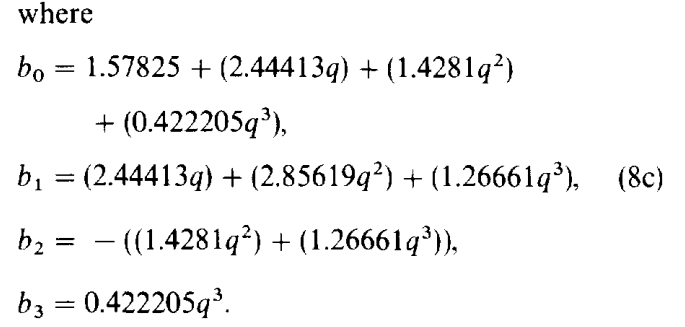q的选择如下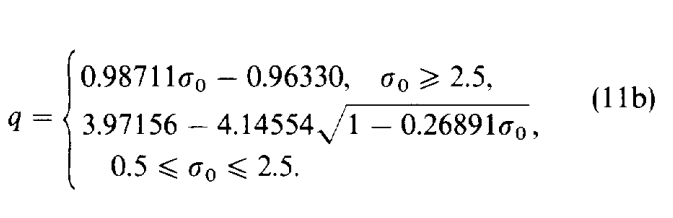## 示例演示

对图像进行高斯滤波而言，假设滤波器半径为r，我们常用的高斯模板则对于图像每个像素的算法复杂度是O(r^2)。高斯滤波器的kernel是可分离的(separable)，也就是说，可以将2D的高斯kernel分解为两个1D的kernel，先沿x方向对图像进行1D高斯kernel的卷积，然后沿y方向对图像进行1D的高斯kernel卷积，最后的结果和使用一个2D高斯kernel对图像卷积效果是一样的。这样一来，针对每个像素，滤波器的算法复杂度降为O(r)。下面实现递归高斯滤波法，即上面第四种方法。完整工程代码。

﻿/**********************************************************************

**********************************************************************/
#include <opencv2/opencv.hpp>
using namespace cv;

typedef struct
{
int    N;
float  sigma;
double B;
double b;
} GaussCoefs;

/*
* Calculate the coefficients for the recursive filter algorithm
* Fast Computation of gaussian blurring.
*/
void ComputeGaussCoefs(GaussCoefs *c, float sigma)
{
/*
* Papers:  "Recursive Implementation of the gaussian filter.",
*          Ian T. Young , Lucas J. Van Vliet, Signal Processing 44, Elsevier 1995.
* formula: 11b       computation of q
*          8c        computation of b0..b1
*          10        alpha is normalization constant B
*/
float q = 0, q2 = 0, q3 = 0;
if (sigma >= 2.5)
{
q = 0.98711 * sigma - 0.96330;
}
else if ((sigma >= 0.5) && (sigma < 2.5))
{
q = 3.97156 - 4.14554 * (float)sqrt((double)1 - 0.26891 * sigma);
}
else
{
q = 0.1147705018520355224609375;
}

q2 = q * q;
q3 = q * q2;
c->b = (1.57825 + (2.44413*q) + (1.4281 *q2) + (0.422205*q3));
c->b = ((2.44413*q) + (2.85619*q2) + (1.26661 *q3));
c->b = (-((1.4281*q2) + (1.26661 *q3)));
c->b = ((0.422205*q3));
c->B = 1.0 - ((c->b + c->b + c->b) / c->b);
c->sigma = sigma;
c->N = 3;
}

void RecursiveGausssmooth(float *in, float *out, int size, int rowstride, GaussCoefs *c)
{
/*
* Papers:  "Recursive Implementation of the gaussian filter.",
*          Ian T. Young , Lucas J. Van Vliet, Signal Processing 44, Elsevier 1995.
* formula: 9a        forward filter
*          9b        backward filter
*          fig7      algorithm
*/
int i, n, bufsize;
float *w1, *w2;

/* forward pass */
bufsize = size + 3;
size -= 1;
w1 = new float[bufsize];
w2 = new float[bufsize];
w1 = in;
w1 = in;
w1 = in;
for (i = 0, n = 3; i <= size; i++, n++)
{
w1[n] = (float)(c->B*in[i*rowstride] +
((c->b * w1[n - 1] +
c->b * w1[n - 2] +
c->b * w1[n - 3]) / c->b));
}

/* backward pass */
w2[size + 1] = w1[size + 3];
w2[size + 2] = w1[size + 3];
w2[size + 3] = w1[size + 3];
for (i = size, n = i; i >= 0; i--, n--)
{
w2[n] = out[i * rowstride] = (float)(c->B*w1[n] +
((c->b * w2[n + 1] +
c->b * w2[n + 2] +
c->b * w2[n + 3]) / c->b));
}

delete[] w1;
delete[] w2;
}

/*
@function: 2D gauss filter
*/
void RecursiveGausssmooth2D(const Mat &src, Mat &dst, float sigma = 2)
{
GaussCoefs c;
ComputeGaussCoefs(&c, sigma);
const int height = src.rows;
const int width = src.cols;
if (src.channels() == 1)
{
Mat srccopy;
src.convertTo(srccopy, CV_32FC1);
Mat dstcopy;
src.convertTo(dstcopy, CV_32FC1);
float* srcdata = srccopy.ptr<float>(0);
float* dstdata = dstcopy.ptr<float>(0);
for (int y = 0; y < height; y++)
{
RecursiveGausssmooth(srcdata + y * width, dstdata + y * width, width, 1, &c);
}
for (int x = 0; x < width; x++)
{
RecursiveGausssmooth(srcdata + x, dstdata + x, height, width, &c);
}
dstcopy.convertTo(dst, src.type());
}
else if (src.channels() == 3)
{
Mat srccopy;
src.convertTo(srccopy, CV_32FC3);
Mat dstcopy;
src.convertTo(dstcopy, CV_32FC3);
float* srcdata = srccopy.ptr<float>(0);
float* dstdata = dstcopy.ptr<float>(0);
for (int y = 0; y < height; y++)
{
RecursiveGausssmooth(srcdata + y * width, dstdata + y * width, width, 3, &c);
RecursiveGausssmooth(srcdata + y * width + 1, dstdata + y * width + 1, width, 3, &c);
RecursiveGausssmooth(srcdata + y * width + 2, dstdata + y * width + 2, width, 3, &c);
}
for (int x = 0; x < width; x++)
{
RecursiveGausssmooth(dstdata + x, dstdata + x, height, width * 3, &c);
RecursiveGausssmooth(dstdata + x + 1, dstdata + x + 1, height, width * 3, &c);
RecursiveGausssmooth(dstdata + x + 2, dstdata + x + 2, height, width * 3, &c);
}
dstcopy.convertTo(dst, src.type());
}
else
return;

}

int main(int argc, char *argv[])
{
imshow("original", src);

Mat dst;
RecursiveGausssmooth2D(src, dst);
imshow(" RecursiveGausssmooth", dst);

waitKey(0);
return EXIT_SUCCESS;
}


## 运行结果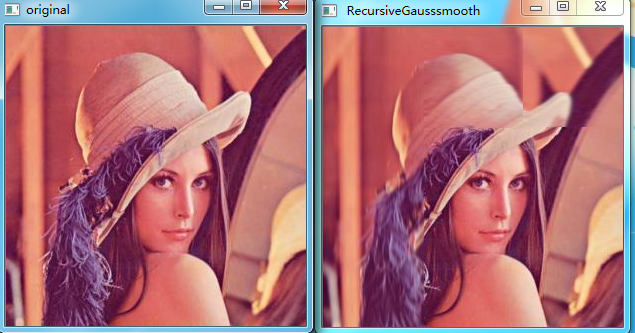2017-10-12 09:25:00 weixin_33670713 阅读数 9
• ###### OpenCV3.2 Java图像处理视频学习教程

OpenCV3.2 Java图像处理视频培训课程：基于OpenCV新版本3.2.0详细讲述Java OpenCV图像处理部分内容，包括Mat对象使用、图像读写、 基于常用核心API讲述基本原理、使用方法、参数、代码演示、图像处理思路与流程讲授。主要内容包括opencv像素操作、滤波、边缘提取、直线与圆检测、形态学操作与分水岭、图像金子塔融合重建、多尺度模板匹配、opencv人脸检测、OpenCV跟Tomcat使用实现服务器端图像处理服务。

4257 人正在学习 去看看 贾志刚

拉普拉斯锐化图像是根据图像某个像素的周围像素到此像素的突变程度有关，也就是说它的依据是图像像素的变化程度。我们知道，一个函数的一阶微分描述了函数图像是朝哪里变化的，即增长或者降低；而二阶微分描述的则是图像变化的速度，急剧增长下降还是平缓的增长下降。那么据此我们可以猜测出依据二阶微分能够找到图像的色素的过渡程度，例如白色到黑色的过渡就是比较急剧的。

或者用官方点的话说：当邻域中心像素灰度低于它所在的领域内其它像素的平均灰度时，此中心像素的灰度应被进一步降低，当邻域中心像素灰度高于它所在的邻域内其它像素的平均灰度时，此中心像素的灰度应被进一步提高，以此实现图像的锐化处理。

运用拉普拉斯可以增强图像的细节，找到图像的边缘。但是有时候会把噪音也给增强了，那么可以在锐化前对图像进行平滑处理。

先看一阶偏微分和推出的二元函数微分：这个成为四邻域也就是上面的二阶微分法这个是八邻域。

【注】从上面的两种模板中就可以看出，如果一个黑色平面中有一个白点，那么模板矩阵可以使这个白点更亮。由于图像边缘就是灰度发生跳变的区域，所以拉普拉斯模板对边缘检测很有用。1 a=imread('yue.jpg');
2 a=rgb2gray(a);
3 %figure,imshow(a);
4 c=[443  602];
5 c=a;
6 for j=2:601
7     c(1,j)=a(1,j-1);
8     c(443,j)=a(441,j-1);
9 end
10 for i=2:442
11     c(i,1)=a(i-1,1);
12     c(i,602)=a(i-1,600);
13 end
14 subplot(1,2,1);
15 imshow(c);
16 for i=2:442
17     for j=2:601
18         lpls=8*c(i,j)-c(i-1,j-1)-c(i-1,j+1)-c(i+1,j-1)-c(i+1,j+1)-c(i-1,j)-c(i+1,j)-c(i,j-1)-c(i,j+1);
19         if lpls<0
20             a(i-1,j-1)=a(i-1,j-1)-lpls; %加上a(i-1,j-1),就是拉普拉斯增强滤波器。
21         else
22             a(i-1,j-1)=a(i-1,j-1)+lpls;
23         end
24     end
25 end
26 subplot(1,2,2);
27 imshow(a);ps：为了使最大值滤波器的效果更直观，我们用一张加了高斯噪声的图片来测试。

 1 a=zeros(500,600);
2 a=imnoise(a,'gaussian',0,0.03);   %将均值0,方差为0.03的高斯噪声加到图像a上
3 subplot(1,3,1);
4 imshow(a);
5 %中值滤波器，调用medfilt函数。
6 b=medfilt2(a,[3,3]);
7 subplot(1,3,2);
8 imshow(b);
9 %最大值滤波器，具体实现原理操作如下。
10 c=[502  602];
11 c=a;
12 for j=2:601
13     c(1,j)=a(1,j-1);
14     c(502,j)=a(500,j-1);
15 end
16 for i=2:501
17     c(i,1)=a(i-1,1);
18     c(i,602)=a(i-1,600);
19 end
20 for i=2:501
21     for j=2:601
22         mx=c(i,j);
23         for x=-1:1
24             for y=-1:1
25                 if mx<c(i+x,j+y)
26                     mx=c(i+x,j+y);
27                 else continue;
28                 end
29             end
30         end
31         a(i-1,j-1)=mx;
32     end
33 end
34 subplot(1,3,3);
35 imshow(a);（ps：我不知道为什么循环写不行，我发现赋给每个像素点的最大值是28，在原图像中明明可以找到比这更大的像素点，但是不知道为什么赋不了，问题仍待解决中，有知道的可以在评论区留言，谢谢~）

 1 a=imread('diqiu.jpg');
2 %subplot(1,3,1);
3 %imshow(a);
4 a=rgb2gray(a);
5 c=filter2(fspecial('average',3),a)/255;
6 % for i=2 : 1199
7 %     for j=2 : 1071
8 %         sum=0;
9 %         for n=-1 : 1
10 %             for m=-1 :1
11 %                 sum=sum+a(i+n ,j+m);
12 %             end
13 %         end
14 %         c(i , j)=sum/9;
15 %     end
16 % end
17 subplot(1,2,2);
18 imshow(c);
19 subplot(1,2,1);
20 imshow(a);http://blog.csdn.net/u012808193/article/details/45722283

a=imread('diqiu.jpg');
a=rgb2gray(a);
figure,imshow(a);
a=double(a);
sobel=edge(a,'Sobel');
roberts=edge(a,'Roberts');
prewitt=edge(a,'Prewitt');
figure,imshow(sobel), title('sobel');
figure,imshow(roberts),title('roberts');
figure,imshow(prewitt),title('prewitt');2019-06-07 16:51:01 jameschen9051 阅读数 314
• ###### OpenCV3.2 Java图像处理视频学习教程

OpenCV3.2 Java图像处理视频培训课程：基于OpenCV新版本3.2.0详细讲述Java OpenCV图像处理部分内容，包括Mat对象使用、图像读写、 基于常用核心API讲述基本原理、使用方法、参数、代码演示、图像处理思路与流程讲授。主要内容包括opencv像素操作、滤波、边缘提取、直线与圆检测、形态学操作与分水岭、图像金子塔融合重建、多尺度模板匹配、opencv人脸检测、OpenCV跟Tomcat使用实现服务器端图像处理服务。

4257 人正在学习 去看看 贾志刚

在《数字图像处理》一书中介绍了用于降低图像噪声的均值滤波器，分别有算数均值滤波器、几何均值滤波器、谐波均值滤波器、逆谐波均值滤波器。除了降噪，均值滤波器也可以模糊图像，滤波器大小为3、5、7...2n+1，滤波器越大计算量越大，产生的图像越模糊。这里采用纯java对几种算法进行实现，代码如下：

import java.awt.image.BufferedImage;

/**
* 几种均值滤波算法实现 1、算术均值滤波 2、几何均值滤波 3、谐波均值滤波 4、逆谐波均值滤波
*
*/
public class AverageFilter {
// singleton
private static AverageFilter averageFilter = new AverageFilter();

public static AverageFilter getInstance() {
return averageFilter;
}

private AverageFilter() {
}

/**
* 算术均值滤波 滤波器大小：param取值3、5、7、9...(2n+1 ) 产生一幅模糊图像
*
* @param image
*/
public void arithmeticAverageFilter(BufferedImage image, int param) {
// 创建一个临时图像，为了保证原图边缘参与计算，临时图像比原图大param-1
BufferedImage tempImage = new BufferedImage(image.getWidth() + param - 1, image.getHeight() + param - 1,
image.getType());
// 对图像进行填充，边缘像素采用最近像素填充
nearFillEdge(tempImage, image, param);
// 进行卷积运算
for (int i = (param - 1) / 2; i < tempImage.getWidth() - (param - 1) / 2; i++) {
for (int j = (param - 1) / 2; j < tempImage.getHeight() - (param - 1) / 2; j++) {
int r = 0, g = 0, b = 0;
// 计算滤波器内所有像素，R、G、B各个分量总和
for (int x = -(param - 1) / 2; x <= (param - 1) / 2; x++) {
for (int y = -(param - 1) / 2; y <= (param - 1) / 2; y++) {
int tempRGB = tempImage.getRGB(i + x, j + y);
int tempR = (tempRGB >> 16) & 0xff;
int tempG = (tempRGB >> 8) & 0xff;
int tempB = tempRGB & 0xff;
r += tempR;
g += tempG;
b += tempB;
}
}
// 采用总和除以滤波器内总像素数量得到均值
r = (int) (r / Math.pow(param, 2));
g = (int) (g / Math.pow(param, 2));
b = (int) (b / Math.pow(param, 2));
int rgb = (255 & 0xff) << 24 | (clamp(r) & 0xff) << 16 | (clamp(g) & 0xff) << 8 | (clamp(b) & 0xff);
image.setRGB(i - (param - 1) / 2, j - (param - 1) / 2, rgb);
}
}
}

/**
* 几何均值滤波
*
* @param image
* @param param
*/
public void geometryAverageFilter(BufferedImage image, int param) {
// 创建临时图像
BufferedImage tempImage = new BufferedImage(image.getWidth() + param - 1, image.getHeight() + param - 1,
image.getType());
// 填充边缘
nearFillEdge(tempImage, image, param);
// 进行卷积运算
for (int i = (param - 1) / 2; i < tempImage.getWidth() - (param - 1) / 2; i++) {
for (int j = (param - 1) / 2; j < tempImage.getHeight() - (param - 1) / 2; j++) {
double r = 1.0, g = 1.0, b = 1.0;
for (int x = -(param - 1) / 2; x <= (param - 1) / 2; x++) {
for (int y = -(param - 1) / 2; y <= (param - 1) / 2; y++) {
int tempRGB = tempImage.getRGB(i + x, j + y);
double tempR = (tempRGB >> 16) & 0xff;
double tempG = (tempRGB >> 8) & 0xff;
double tempB = tempRGB & 0xff;
r *= Math.pow(tempR + 1, 1.0 / (param * param));
g *= Math.pow(tempG + 1, 1.0 / (param * param));
b *= Math.pow(tempB + 1, 1.0 / (param * param));
}
}
int rgb = (255 & 0xff) << 24 | (clamp((int) r) & 0xff) << 16 | (clamp((int) g) & 0xff) << 8
| (clamp((int) b) & 0xff);
image.setRGB(i - (param - 1) / 2, j - (param - 1) / 2, rgb);
}
}
}

/**
* 谐波均值滤波
*
* @param image
* @param param
*/
public void harmonicFilter(BufferedImage image, int param) {
// 创建temp图像
BufferedImage tempImage = new BufferedImage(image.getWidth() + param - 1, image.getHeight() + param - 1,
image.getType());
// 填充边缘
nearFillEdge(tempImage, image, param);
// 进行卷积运算
for (int i = (param - 1) / 2; i < tempImage.getWidth() - (param - 1) / 2; i++) {
for (int j = (param - 1) / 2; j < tempImage.getHeight() - (param - 1) / 2; j++) {
double r = 0, g = 0, b = 0;
for (int x = -(param - 1) / 2; x <= (param - 1) / 2; x++) {
for (int y = -(param - 1) / 2; y <= (param - 1) / 2; y++) {
int tempRGB = tempImage.getRGB(i + x, j + y);
double tempR = (tempRGB >> 16) & 0xff;
double tempG = (tempRGB >> 8) & 0xff;
double tempB = tempRGB & 0xff;
r += 1 / tempR;
g += 1 / tempG;
b += 1 / tempB;
}
}
r = param * param / r;
g = param * param / g;
b = param * param / b;
int rgb = (255 & 0xff) << 24 | (clamp((int) r) & 0xff) << 16 | (clamp((int) g) & 0xff) << 8
| (clamp((int) b) & 0xff);
image.setRGB(i - (param - 1) / 2, j - (param - 1) / 2, rgb);
}
}
}

/**
* 逆谐波均值滤波
*
* @param image
* @param param
* @param Q
*            当Q=0为算术均值滤波，Q=-1为谐波均值滤波；Q为正，消除胡椒噪声，Q为负，消除盐粒噪声
*/
public void reverseHarmonicFilter(BufferedImage image, int param, int Q) {
// 创建temp图像
BufferedImage tempImage = new BufferedImage(image.getWidth() + param - 1, image.getHeight() + param - 1,
image.getType());
// 填充边缘
nearFillEdge(tempImage, image, param);
// 进行卷积运算
for (int i = (param - 1) / 2; i < tempImage.getWidth() - (param - 1) / 2; i++) {
for (int j = (param - 1) / 2; j < tempImage.getHeight() - (param - 1) / 2; j++) {
double r = 0, g = 0, b = 0, r1 = 0, g1 = 0, b1 = 0;
for (int x = -(param - 1) / 2; x <= (param - 1) / 2; x++) {
for (int y = -(param - 1) / 2; y <= (param - 1) / 2; y++) {
int tempRGB = tempImage.getRGB(i + x, j + y);
double tempR = (tempRGB >> 16) & 0xff;
double tempG = (tempRGB >> 8) & 0xff;
double tempB = tempRGB & 0xff;
r += Math.pow(tempR, Q + 1);
g += Math.pow(tempG, Q + 1);
b += Math.pow(tempB, Q + 1);
r1 += Math.pow(tempR, Q);
g1 += Math.pow(tempG, Q);
b1 += Math.pow(tempB, Q);
}
}
r = r / r1;
g = g / g1;
b = b / b1;
int rgb = (255 & 0xff) << 24 | (clamp((int) r) & 0xff) << 16 | (clamp((int) g) & 0xff) << 8
| (clamp((int) b) & 0xff);
image.setRGB(i - (param - 1) / 2, j - (param - 1) / 2, rgb);
}
}

}

// 判断r,g,b值，大于256返回256，小于0则返回0,0到256之间则直接返回原始值
private int clamp(int rgb) {
if (rgb > 255)
return 255;
if (rgb < 0)
return 0;
return rgb;
}

// 填充图像边缘空白像素,使用最近像素填充
private void nearFillEdge(BufferedImage tempImage, BufferedImage image, int param) {
for (int i = 0; i < tempImage.getWidth(); i++) {
for (int j = 0; j < tempImage.getHeight(); j++) {
// 临时图像位置没超过原图第一个位置,左下角
if (i <= (param - 1) / 2 & j <= (param - 1) / 2) {
int rgb = image.getRGB(0, 0);
tempImage.setRGB(i, j, rgb);
}
// 临时图像位置超过横坐标最大，小于纵坐标最小，右下角
if (i >= tempImage.getWidth() - (param - 1) / 2 - 1 & j <= (param - 1) / 2) {
int rgb = image.getRGB(image.getWidth() - 1, 0);
tempImage.setRGB(i, j, rgb);
}
// 临时图像位置超过纵坐标最大，小于横坐标最小，左上角
if (j >= tempImage.getHeight() - (param - 1) / 2 - 1 & i <= (param - 1) / 2) {
int rgb = image.getRGB(0, image.getHeight() - 1);
tempImage.setRGB(i, j, rgb);
}
// 临时图像位置横纵坐标都超过原图最大位置，右上角
if (i >= tempImage.getWidth() - (param - 1) / 2 - 1
& j >= tempImage.getHeight() - (param - 1) / 2 - 1) {
int rgb = image.getRGB(image.getWidth() - 1, image.getHeight() - 1);
tempImage.setRGB(i, j, rgb);
}
// 临时图像位置横坐标大于最小，小于最大，纵坐标小于最小，正下方
if (i > (param - 1) / 2 & i < tempImage.getWidth() - (param - 1) / 2 - 1 & j <= (param - 1) / 2) {
int rgb = image.getRGB(i - (param - 1) / 2, 0);
tempImage.setRGB(i, j, rgb);
}
// 临时图像位置横坐标小于最小，纵坐标大于最小，小于最大，左边
if (j > (param - 1) / 2 & j < tempImage.getHeight() - (param - 1) / 2 - 1 & i <= (param - 1) / 2) {
int rgb = image.getRGB(0, j - (param - 1) / 2);
tempImage.setRGB(i, j, rgb);
}
// 右边
if (j > (param - 1) / 2 & j < tempImage.getHeight() - (param - 1) / 2 - 1
& i >= tempImage.getWidth() - (param - 1) / 2 - 1) {
int rgb = image.getRGB(image.getWidth() - 1, j - (param - 1) / 2);
tempImage.setRGB(i, j, rgb);
}
// 上方
if (i > (param - 1) / 2 & i < tempImage.getWidth() - (param - 1) / 2 - 1
& j >= tempImage.getHeight() - (param - 1) / 2 - 1) {
int rgb = image.getRGB(i - (param - 1) / 2, image.getHeight() - 1);
tempImage.setRGB(i, j, rgb);
}
// 中间
if (i > (param - 1) / 2 & i < tempImage.getWidth() - (param - 1) / 2 - 1 & j > (param - 1) / 2
& j < tempImage.getHeight() - (param - 1) / 2 - 1) {
int rgb = image.getRGB(i - (param - 1) / 2, j - (param - 1) / 2);
tempImage.setRGB(i, j, rgb);
}
}
}
}
}


import java.awt.image.BufferedImage;
import java.io.File;

import javax.imageio.ImageIO;

public class test {
public static void main(String[] args) throws Exception{
File input = new File("C:/桌面/AverageFilter/1.jpg");
File output = new File("C:/桌面/AverageFilter/2.jpg");
AverageFilter.getInstance().reverseHarmonicFilter(image, 3, 2);
ImageIO.write(image, "jpg", output);
}
}


2016-10-25 22:02:08 wzmsltw 阅读数 12097
• ###### OpenCV3.2 Java图像处理视频学习教程

OpenCV3.2 Java图像处理视频培训课程：基于OpenCV新版本3.2.0详细讲述Java OpenCV图像处理部分内容，包括Mat对象使用、图像读写、 基于常用核心API讲述基本原理、使用方法、参数、代码演示、图像处理思路与流程讲授。主要内容包括opencv像素操作、滤波、边缘提取、直线与圆检测、形态学操作与分水岭、图像金子塔融合重建、多尺度模板匹配、opencv人脸检测、OpenCV跟Tomcat使用实现服务器端图像处理服务。

4257 人正在学习 去看看 贾志刚

### 椒盐噪声

void salt(Mat &image, float salt_ratio){
int n=image.rows*image.cols*salt_ratio;
for (int k = 0; k < n; k++)
{
int i = rand() % image.cols;  //cols 和 rows 给出图像的宽与高
int j = rand() % image.rows;
int type= rand() %2;
if (type==1){
image.at<Vec3b>(j, i) = 255;
image.at<Vec3b>(j, i) = 255;
image.at<Vec3b>(j, i) = 255;
}
else{
image.at<Vec3b>(j, i) = 0;
image.at<Vec3b>(j, i) = 0;
image.at<Vec3b>(j, i) = 0;
}
}
}### 滤波器原理

#### 算术均值滤波器

f^(x,y)=1mn(s,t)Sxyg(s,t)

#### 几何均值滤波器

f^(x,y)=(s,t)Sxyg(s,t)1mn

#### 谐波滤波器

f^(x,y)=mn(s,t)Sxy1g(s,t)

#### 中值滤波器

f^(x,y)=median(s,t)Sxy(g(s,t))

### 编程实现

根据以上公式，实现了四种模板大小可变的滤波器。
主要编程思想为：构建一个ImageRecovery类，其中filter函数为公用的滤波器接口，在filter函数中对result（Mat格式3通道图片）进行遍历，然后根据选择的滤波器类型调用不同的滤波器模板，进行滤波运算。滤波器模板函数则作为类的private函数。
此外，在谐波滤波和几何滤波中，若模板中有一个像素的取值为0，则不将这个点引入计算。若引入计算则会导致错误或是0像素点相邻的一片区域完全变黑。相当于增加了图像的噪声。代码如下：

#include "stdafx.h"
#include <opencv2/core/core.hpp>
#include <opencv2/highgui/highgui.hpp>
#include <opencv2/opencv.hpp>

using namespace cv;
using namespace std;

class ImageRecovery{
private:
double filter_aver(Mat src)
{
//算术均值滤波
double sum=0;
for (int i =0;i < src.rows;i++ ){
uchar* data=src.ptr<uchar>(i);
for (int j =0;j <src.cols;j++){
sum+=double(data[j]);
}
}
return sum/double(src.cols* src.rows);
}
double filter_geo(Mat src)
{
//几何均值滤波
double geo=1;
for (int i =0;i < src.rows;i++ ){
uchar* data=src.ptr<uchar>(i);
for (int j =0;j <src.cols;j++){
if (data[j]!=0) geo*=data[j];
}
}
double power=1.0/double(src.cols*src.rows);
return pow(geo,power);
}
double filter_har(Mat src)
{
//谐波滤波
double har=0;
for (int i =0;i < src.rows;i++ ){
uchar* data=src.ptr<uchar>(i);
for (int j =0;j <src.cols;j++){
if (data[j]!=0) har+=1/(double)(data[j]);
}
}
return (src.cols*src.rows)/har;
}
void BubbleSort(float* pData, int count)
{
//冒泡排序，用于中值滤波
float tData;
for (int i = 1; i < count; i++){
for (int j = count - 1; j >= i; j--){
if (pData[j] < pData[j - 1]){
tData = pData[j - 1];
pData[j - 1] = pData[j];
pData[j] = tData;
}
}
}
}
double filter_median(Mat src)
{
//中值滤波
int index=0;
int bubble_len=(src.cols)*(src.rows);
float* bubble=new float[bubble_len];
for (int i =0;i < src.rows;i++ ){
uchar* data=src.ptr<uchar>(i);
for (int j =0;j <src.cols;j++){
bubble[index] = data[j];
index ++;
}
}
BubbleSort(bubble,bubble_len);
double median=bubble[bubble_len/2];
return median;
}
public:
void salt(Mat &image, float salt_ratio )
{
//salt_ratio为加入椒盐噪声的比例
int n=image.rows*image.cols*salt_ratio;
for (int k = 0; k < n; k++)
{
int i = rand() % image.cols;
int j = rand() % image.rows;
int type= rand() %2;
if (type==1){
image.at<Vec3b>(j, i) = 255;
image.at<Vec3b>(j, i) = 255;
image.at<Vec3b>(j, i) = 255;
}
else{
image.at<Vec3b>(j, i) = 0;
image.at<Vec3b>(j, i) = 0;
image.at<Vec3b>(j, i) = 0;
}
}
}
Mat filter(Mat image,string filter_type,Size size)
{
//image为输入待滤波图像，filter_tpye为滤波器类型，size为滤波器的尺寸
Mat result;
image.copyTo(result);
Mat channel;
split(image,channel);
int l =(size.height-1)/2;
int w =(size.width-1)/2;
for (int i = l;i < result.rows-l;i ++){
for (int j =w;j < result.cols-w;j ++){
for (int ii =0;ii < 3;ii++){
if (filter_type=="aver")    result.at<Vec3b>(i,j)[ii]=saturate_cast<uchar>(filter_aver(channel[ii](Rect(j-w,i-l,size.width,size.height))));
if (filter_type=="geo" )    result.at<Vec3b>(i,j)[ii]=saturate_cast<uchar>(filter_geo(channel[ii](Rect(j-w,i-l,size.width,size.height))));
if (filter_type=="har" )    result.at<Vec3b>(i,j)[ii]=saturate_cast<uchar>(filter_har(channel[ii](Rect(j-w,i-l,size.width,size.height))));
if (filter_type=="median" ) result.at<Vec3b>(i,j)[ii]=saturate_cast<uchar>(filter_median(channel[ii](Rect(j-w,i-l,size.width,size.height))));
}
}
}
return result;
}
};

int main()
{
//初始化IR类
ImageRecovery IR;
//加入椒盐噪声
IR.salt(img,0.1);
imshow("salt",img);
//对噪声图片进行滤波
Mat result=IR.filter(img,"geo",Size(3,3));
imshow("result",result);
waitKey();
return 0;
}总的来看，基于个人主观的判断，我认为对于椒盐噪声图片的恢复，中值滤波的效果最好，谐波滤波的效果次之，均值滤波的效果再次，几何滤波的效果最差。
而对于滤波器的不同模板大小，从结果上可以看出选择更大的模板可以使得结果图像中的噪声更加平滑，但同时图像也变得模糊了。这是由于更大的滤波器模板使得图像整体变得平滑了。

这次也是我第一次使用markdown写博客，线下使用wiznote的markdown模式进行编辑，图片则存储在七牛云上。这样在线下写完的博客可以直接复制到csdn上，省去了我之前每次上传博客还要改格式以及传图片的烦恼。此外，用markdown写感觉格式上也好看了很多，特别是公式方面。